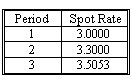### CFA Practice Question

There are 490 practice questions for this study session.

### CFA Practice Question

Given the following table of spot rates, calculate a zero-volatility spread for a three-period instrument with a 7.5% coupon priced at \$100.70.A. 350 basis points
B. 330 basis points
C. 250 basis points

\$100.70 = 3.75/(1+(.03+zs)/2)1 + 3.75/(1+(.033+zs)/2)2 + 103.75/(1+(.035053+zs)/2)3
Using trial and error, the value of zs = 350 basis points.

User Comment
quincy How to use Calculator for this one?
cuong pick one option and then put into the equation. Using trial and error to get the final result.
haarlemmer The problem here is the tech is so much advanced that using computer would be much easier. Maybe someday, we will have our exam on computer rather with calculator.
danlan Suppose it's semiannual coupon, so the coupon for a period is 3.75 instead of 7.5
myanmar but the spot rates are on an annual basis, right?
don't we have to devide the spotrates by 2
myanmar ok silly question, i've not seen the "/2"
cwrolfe Calculation of z-spread is not in the LOS
valeris Computing using calculator:

1. For each of the three periods determine PV of cash flow using spot rates (coupon=3.75) :

a) Period 1 - 3.69458
b) Period 2 - 3.62925
c) Period 3 - 98.4807

Sum them up to get PV = 105.80448

Calculate the rate using PV above, FV =100, PMT = 3.75, N = 3.

Rate = 1.747 x 2 = 3.5%
Bevin I just put in

PV = -100.7
FV = 100
N = 3
PMT = 3.75

Then CPT I/Y = 3.5
thanks I think valeris way of calculating rate is ok.. not sure abt Bevin's... esp because u used semi-annual rate for pmt and so technically 1/Y would also be semi-annual. Thus if you multiply 3.5*2, then the rate is no longer correct.
MFTIOA Bevin - need to calculate the cash flow for each spot rate... that's the definition of the z-spread
mishis valeris has N wrong, it should be 6 periods in calc. How to calculate this without using trial and error?
TheHTrader The trial and error approach is conceptually better understood but time-consuming. I agree to valeris - easier to calculate but need a little adjustment in thinking it through - the last step integrates bootstrapping in PV = "purchase price", and the yield generated should be the z-spread.
alallstar Valeris seems to have it right.
mishis: Why would you use 6 periods? It says 3 periods, not 3 years.
hardig Help - how did valeris get the pv cashflows - I can't get it to work out on BA ii+
gulfa99 guys its a simple question. You can answer it by looking at the options. Look at the term structure of spot rates, they are upward slopping. As we have learned earlier in a upward slopping spot curve, z rates are always higher than spot rates. By this logic answer is A, all other options are lower.
johntan1979 Ignore gulfa99's comment. A is not higher than period 3's spot rate of 3.5053%.

Follow valeris' way. That's the fastest and correct way.
Shaan23 Valeris -- Impressive

Would not have thought of that.
MrFortei Please can someone tell how Valeris got his period a,b, and c answer using the ba ii plus calculator?